GeeksforGeeks App
Open AppBrowser
Continue

## Related Articles

• Write an Interview Experience
• Mathematical Algorithms

# Sieve of Eratosthenes

Given a number n, print all primes smaller than or equal to n. It is also given that n is a small number.

Example:

Input : n =10
Output : 2 3 5 7

Input : n = 20
Output: 2 3 5 7 11 13 17 19

The sieve of Eratosthenes is one of the most efficient ways to find all primes smaller than n when n is smaller than 10 million or so (Ref Wiki).

Following is the algorithm to find all the prime numbers less than or equal to a given integer n by the Eratosthene’s method:
When the algorithm terminates, all the numbers in the list that are not marked are prime.

Explanation with Example:

Let us take an example when n = 50. So we need to print all prime numbers smaller than or equal to 50.

We create a list of all numbers from 2 to 50.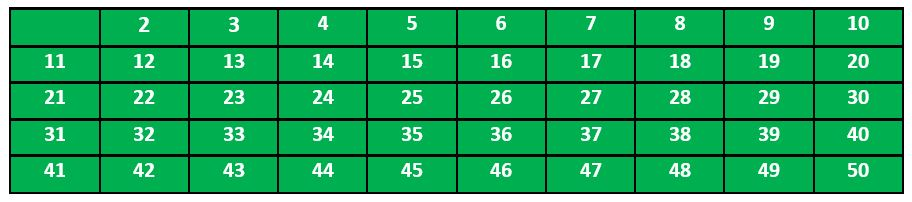According to the algorithm we will mark all the numbers which are divisible by 2 and are greater than or equal to the square of it.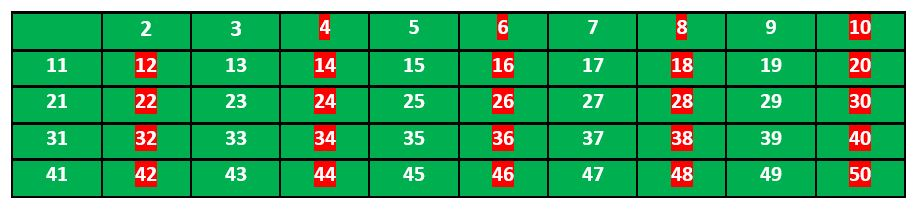Now we move to our next unmarked number 3 and mark all the numbers which are multiples of 3 and are greater than or equal to the square of it.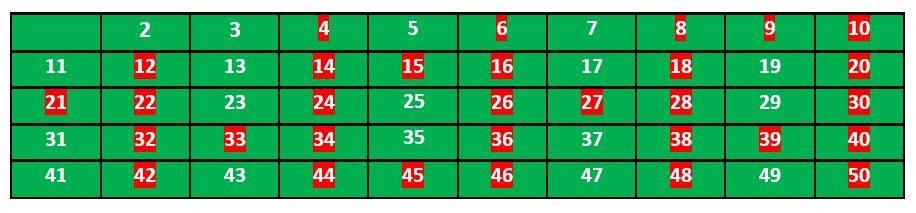We move to our next unmarked number 5 and mark all multiples of 5 and are greater than or equal to the square of it.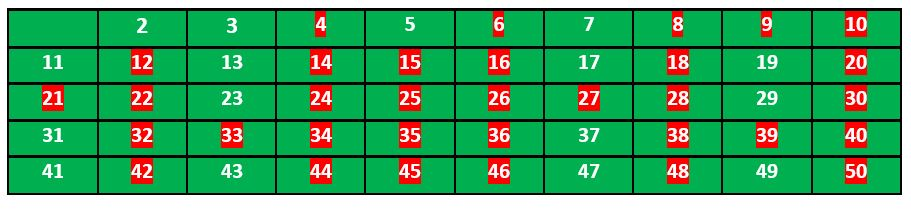We continue this process and our final table will look like below: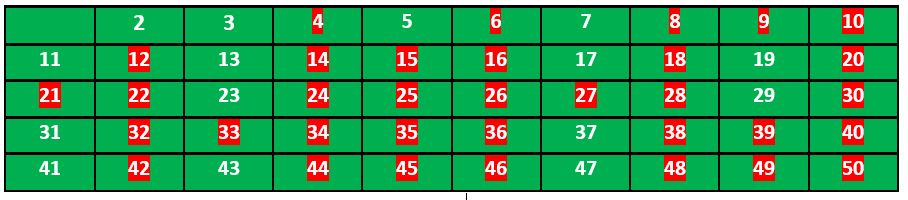So the prime numbers are the unmarked ones: 2, 3, 5, 7, 11, 13, 17, 19, 23, 29, 31, 37, 41, 43, 47.

Thanks to Krishan Kumar for providing the above explanation.

Implementation:

Following is the implementation of the above algorithm. In the following implementation, a boolean array arr[] of size n is used to mark multiples of prime numbers.

## C++

 `// C++ program to print all primes smaller than or equal to``// n using Sieve of Eratosthenes``#include ``using` `namespace` `std;`` ` `void` `SieveOfEratosthenes(``int` `n)``{``    ``// Create a boolean array "prime[0..n]" and initialize``    ``// all entries it as true. A value in prime[i] will``    ``// finally be false if i is Not a prime, else true.``    ``bool` `prime[n + 1];``    ``memset``(prime, ``true``, ``sizeof``(prime));`` ` `    ``for` `(``int` `p = 2; p * p <= n; p++) {``        ``// If prime[p] is not changed, then it is a prime``        ``if` `(prime[p] == ``true``) {``            ``// Update all multiples of p greater than or``            ``// equal to the square of it numbers which are``            ``// multiple of p and are less than p^2 are``            ``// already been marked.``            ``for` `(``int` `i = p * p; i <= n; i += p)``                ``prime[i] = ``false``;``        ``}``    ``}`` ` `    ``// Print all prime numbers``    ``for` `(``int` `p = 2; p <= n; p++)``        ``if` `(prime[p])``            ``cout << p << ``" "``;``}`` ` `// Driver Code``int` `main()``{``    ``int` `n = 30;``    ``cout << ``"Following are the prime numbers smaller "``         ``<< ``" than or equal to "` `<< n << endl;``    ``SieveOfEratosthenes(n);``    ``return` `0;``}`

## C

 `// C program to print all primes smaller than or equal to``// n using Sieve of Eratosthenes``#include ``#include ``#include `` ` `void` `SieveOfEratosthenes(``int` `n)``{``   ` `    ``// Create a boolean array "prime[0..n]" and initialize``    ``// all entries it as true. A value in prime[i] will``    ``// finally be false if i is Not a prime, else true.``    ``bool` `prime[n + 1];``    ``memset``(prime, ``true``, ``sizeof``(prime));`` ` `    ``for` `(``int` `p = 2; p * p <= n; p++) {``        ``// If prime[p] is not changed, then it is a prime``        ``if` `(prime[p] == ``true``) {``            ``// Update all multiples of p greater than or``            ``// equal to the square of it numbers which are``            ``// multiple of p and are less than p^2 are``            ``// already been marked.``            ``for` `(``int` `i = p * p; i <= n; i += p)``                ``prime[i] = ``false``;``        ``}``    ``}`` ` `    ``// Print all prime numbers``    ``for` `(``int` `p = 2; p <= n; p++)``        ``if` `(prime[p])``            ``printf``(``"%d "``,p);``}`` ` `// Driver Code``int` `main()``{``    ``int` `n = 30;``    ``printf``(``"Following are the prime numbers smaller than or equal to %d \n"``, n);``    ``SieveOfEratosthenes(n);``    ``return` `0;``}`` ` `// This code is contributed by Aditya Kumar (adityakumar129)`

## Java

 `// Java program to print all primes smaller than or equal to``// n using Sieve of Eratosthenes`` ` `class` `SieveOfEratosthenes {``    ``void` `sieveOfEratosthenes(``int` `n)``    ``{``        ``// Create a boolean array "prime[0..n]" and``        ``// initialize all entries it as true. A value in``        ``// prime[i] will finally be false if i is Not a``        ``// prime, else true.``        ``boolean` `prime[] = ``new` `boolean``[n + ``1``];``        ``for` `(``int` `i = ``0``; i <= n; i++)``            ``prime[i] = ``true``;`` ` `        ``for` `(``int` `p = ``2``; p * p <= n; p++) {``            ``// If prime[p] is not changed, then it is a``            ``// prime``            ``if` `(prime[p] == ``true``) {``                ``// Update all multiples of p greater than or``                ``// equal to the square of it numbers which``                ``// are multiple of p and are less than p^2``                ``// are already been marked.``                ``for` `(``int` `i = p * p; i <= n; i += p)``                    ``prime[i] = ``false``;``            ``}``        ``}`` ` `        ``// Print all prime numbers``        ``for` `(``int` `i = ``2``; i <= n; i++) {``            ``if` `(prime[i] == ``true``)``                ``System.out.print(i + ``" "``);``        ``}``    ``}`` ` `    ``// Driver Code``    ``public` `static` `void` `main(String args[])``    ``{``        ``int` `n = ``30``;``        ``System.out.print(``"Following are the prime numbers "``);``        ``System.out.println(``"smaller than or equal to "` `+ n);``        ``SieveOfEratosthenes g = ``new` `SieveOfEratosthenes();``        ``g.sieveOfEratosthenes(n);``    ``}``}`` ` `// This code is contributed by Aditya Kumar (adityakumar129)`

## Python3

 `# Python program to print all``# primes smaller than or equal to``# n using Sieve of Eratosthenes`` ` ` ` `def` `SieveOfEratosthenes(n):`` ` `    ``# Create a boolean array``    ``# "prime[0..n]" and initialize``    ``#  all entries it as true.``    ``# A value in prime[i] will``    ``# finally be false if i is``    ``# Not a prime, else true.``    ``prime ``=` `[``True` `for` `i ``in` `range``(n``+``1``)]``    ``p ``=` `2``    ``while` `(p ``*` `p <``=` `n):`` ` `        ``# If prime[p] is not``        ``# changed, then it is a prime``        ``if` `(prime[p] ``=``=` `True``):`` ` `            ``# Update all multiples of p``            ``for` `i ``in` `range``(p ``*` `p, n``+``1``, p):``                ``prime[i] ``=` `False``        ``p ``+``=` `1`` ` `    ``# Print all prime numbers``    ``for` `p ``in` `range``(``2``, n``+``1``):``        ``if` `prime[p]:``            ``print``(p)`` ` ` ` `# Driver code``if` `__name__ ``=``=` `'__main__'``:``    ``n ``=` `20``    ``print``(``"Following are the prime numbers smaller"``),``    ``print``(``"than or equal to"``, n)``    ``SieveOfEratosthenes(n)`

## C#

 `// C# program to print all primes``// smaller than or equal to n``// using Sieve of Eratosthenes``using` `System;`` ` `namespace` `prime {``public` `class` `GFG {`` ` `    ``public` `static` `void` `SieveOfEratosthenes(``int` `n)``    ``{`` ` `        ``// Create a boolean array ``        ``// "prime[0..n]" and``        ``// initialize all entries``        ``// it as true. A value in``        ``// prime[i] will finally be ``        ``// false if i is Not a``        ``// prime, else true.`` ` `        ``bool``[] prime = ``new` `bool``[n + 1];`` ` `        ``for` `(``int` `i = 0; i <= n; i++)``            ``prime[i] = ``true``;`` ` `        ``for` `(``int` `p = 2; p * p <= n; p++) ``        ``{``            ``// If prime[p] is not changed,``            ``// then it is a prime``            ``if` `(prime[p] == ``true``)``            ``{``                ``// Update all multiples of p``                ``for` `(``int` `i = p * p; i <= n; i += p)``                    ``prime[i] = ``false``;``            ``}``        ``}`` ` `        ``// Print all prime numbers``        ``for` `(``int` `i = 2; i <= n; i++)``        ``{``            ``if` `(prime[i] == ``true``)``                ``Console.Write(i + ``" "``);``        ``}``    ``}`` ` `    ``// Driver Code``    ``public` `static` `void` `Main()``    ``{``        ``int` `n = 30;``        ``Console.WriteLine(``            ``"Following are the prime numbers"``);``        ``Console.WriteLine(``"smaller than or equal to "` `+ n);``        ``SieveOfEratosthenes(n);``    ``}``}``}`` ` `// This code is contributed by Sam007.`

## PHP

 ``

## Javascript

 ``

Output

```Following are the prime numbers smaller  than or equal to 30
2 3 5 7 11 13 17 19 23 29 ```

Time Complexity: O(n*log(log(n)))
Auxiliary Space: O(n)

## C++

 `// the following implementation``// stores only halves of odd numbers``// the algorithm is a faster by some constant factors`` ` `#include ``#include ``using` `namespace` `std;`` ` `bitset<500001> Primes;``void` `SieveOfEratosthenes(``int` `n)``{``    ``Primes = 1;``    ``for` `(``int` `i = 3; i*i <= n; i += 2) {``        ``if` `(Primes[i / 2] == 0) {``            ``for` `(``int` `j = 3 * i; j <= n; j += 2 * i)``                ``Primes[j / 2] = 1;``        ``}``    ``}``}`` ` `int` `main()``{``    ``int` `n = 100;``    ``SieveOfEratosthenes(n);``    ``for` `(``int` `i = 1; i <= n; i++) {``        ``if` `(i == 2)``            ``cout << i << ``' '``;``        ``else` `if` `(i % 2 == 1 && Primes[i / 2] == 0)``            ``cout << i << ``' '``;``    ``}``    ``return` `0;``}`

## Java

 `// Java program for the above approach``import` `java.io.*;``public` `class` `GFG {`` ` `  ``static` `int``[] Primes = ``new` `int``[``500001``];`` ` `  ``static` `void` `SieveOfEratosthenes(``int` `n)``  ``{``    ``Primes[``0``] = ``1``;``    ``for` `(``int` `i = ``3``; i * i <= n; i += ``2``) {``      ``if` `(Primes[i / ``2``] == ``0``) {``        ``for` `(``int` `j = ``3` `* i; j <= n; j += ``2` `* i)``          ``Primes[j / ``2``] = ``1``;``      ``}``    ``}``  ``}`` ` `  ``// Driver Code``  ``public` `static` `void` `main(String[] args)``  ``{`` ` `    ``int` `n = ``100``;``    ``SieveOfEratosthenes(n);``    ``for` `(``int` `i = ``1``; i <= n; i++) {``      ``if` `(i == ``2``)``        ``System.out.print(i + ``" "``);``      ``else` `if` `(i % ``2` `== ``1` `&& Primes[i / ``2``] == ``0``)``        ``System.out.print(i + ``" "``);``    ``}``  ``}``}`` ` `// This code is contributed by ukasp.`

## Python3

 `# Python program for the above approach``Primes ``=` `[``0``] ``*` `500001``def` `SieveOfEratosthenes(n) :``     ` `    ``Primes[``0``] ``=` `1``    ``i ``=` `3``    ``while``(i``*``i <``=` `n) : ``        ``if` `(Primes[i ``/``/` `2``] ``=``=` `0``) :``            ``for` `j ``in` `range``(``3` `*` `i, n``+``1``, ``2` `*` `i) : ``                ``Primes[j ``/``/` `2``] ``=` `1``                 ` `        ``i ``+``=` `2``         ` `# Driver Code``if` `__name__ ``=``=` `"__main__"``:`` ` `    ``n ``=` `100``    ``SieveOfEratosthenes(n)``    ``for` `i ``in` `range``(``1``, n``+``1``) :``        ``if` `(i ``=``=` `2``) :``            ``print``( i, end ``=` `" "``)``        ``elif` `(i ``%` `2` `=``=` `1` `and` `Primes[i ``/``/` `2``] ``=``=` `0``) :``            ``print``( i, end ``=` `" "``)``     ` `    ``# This code is contributed by code_hunt.`

## C#

 `// C# program for the above approach``using` `System;``public` `class` `GFG {`` ` `  ``static` `int``[] Primes = ``new` `int``;`` ` `  ``static` `void` `SieveOfEratosthenes(``int` `n)``  ``{``    ``Primes = 1;``    ``for` `(``int` `i = 3; i*i <= n; i += 2) {``      ``if` `(Primes[i / 2] == 0) {``        ``for` `(``int` `j = 3 * i; j <= n; j += 2 * i)``          ``Primes[j / 2] = 1;``      ``}``    ``}``  ``}`` ` `  ``// Driver Code``  ``public` `static` `void` `Main(String[] args) {`` ` `    ``int` `n = 100;``    ``SieveOfEratosthenes(n);``    ``for` `(``int` `i = 1; i <= n; i++) {``      ``if` `(i == 2)``        ``Console.Write(i + ``" "``);``      ``else` `if` `(i % 2 == 1 && Primes[i / 2] == 0)``        ``Console.Write(i + ``" "``);``    ``}``  ``}``}`` ` `// This code is contributed by sanjoy_62.`

## Javascript

 `// A JavaScript Program ``// the following implementation``// stores only halves of odd numbers``// the algorithm is a faster by some constant factors`` ` `let Primes = ``new` `Array(500001).fill(0);`` ` `function` `SieveOfEratosthenes(n)``{``    ``Primes = 1;``    ``for` `(let i = 3; i*i <= n; i += 2) {``        ``let flr = Math.floor(i / 2);``        ``if` `(Primes[flr] == 0) {``            ``for` `(let j = 3 * i; j <= n; j += 2 * i){``                 ``Primes[flr] = 1;``            ``}``        ``}``    ``}``}`` ` `let n = 100;``SieveOfEratosthenes(n);``let res = ``""``;``for` `(let i = 1; i <= n; i++) {``    ``let flr = Math.floor(i / 2);``    ``if` `(i == 2){``        ``res = res + i + ``" "``;``    ``}``    ``else` `if` `(i % 2 == 1 && Primes[flr] == 0){``        ``res = res + i + ``" "``;``    ``}``}``console.log(res);`` ` `// The code is contributed by Gautam goel (gautamgoel962)`

Output

`2 3 5 7 11 13 17 19 23 29 31 37 41 43 47 53 59 61 67 71 73 79 83 89 97 `

Time Complexity: O(n*log(log(n)))
Auxiliary Space: O(n)

You may also like to see :

My Personal Notes arrow_drop_up